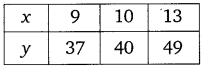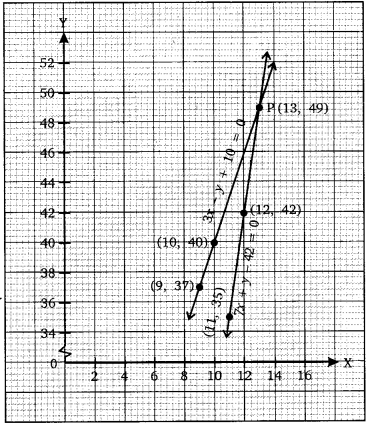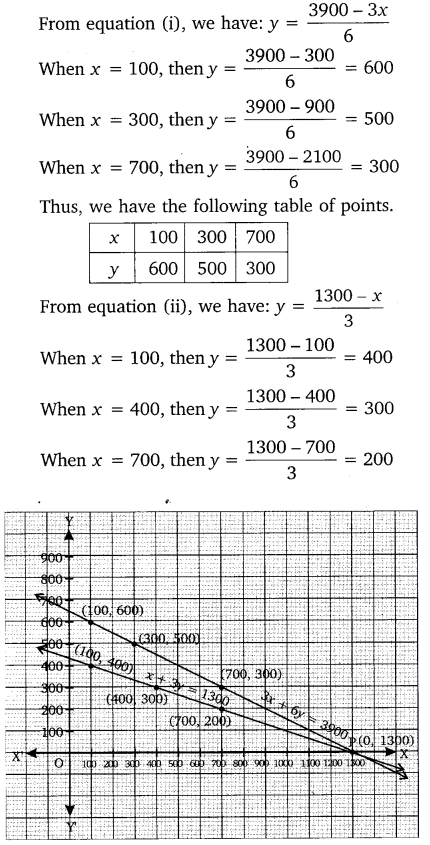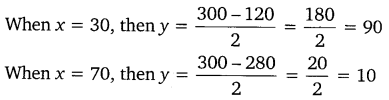# CLASS 10 MATH NCERT SOLUTIONS FOR CHAPTER – 3 Pair of Linear Equations in Two Variables Ex – 3.1

## Pair of Linear Equations in Two Variables

Question 1.
Aftab tells his daughter, “Seven years ago, I was seven times as old as you were then. Also, three years from now, I shall be three times as old as you will be”. Isn’t this interesting? Represent this situation algebraically and graphically.

Solution:
Let the present age of Aftab’s daughter be x years and that of Aftab be y years.
Then, seven years ago:
Aftab’s age was (y – 7) years
and his daughter’s age was (x – 7) years.
According to the first condition:
y – 7 = 7(x – 7)
⇒   y – 7 = 7x – 49
⇒ 7x – y = 42    …(i)
Five years later:
Aftab’s age will be (y + 5) years,
and his daughter’s age will be (x + 5) years
According to the second condition:
y + 5 = 3(x + 5)
⇒  y + 5 = 3x + 15
⇒ 3x – y = -10    …(ii)
For graphical representation:
From equation (i), we have:
y = 7x – 42
When x = 11, then y = 7 x 11 – 42 = 35
When x = 12, then y = 7 x 12 – 42 = 42
When x = 13, then y = 7 x 13 – 42 = 49
Thus, we have the following table of points:From equation (ii), we have:
y = 3x + 10
When x = 9, then y = 3 x 9 + 10 = 37
When x = 10, then y = 3 x 10 + 10 = 40
When x = 13, then y = 3 x 13 + 10 = 49
Thus, we have the following table of points:Plotting the points of each table, we obtain the required graph of two intersecting lines.Question 2.
The coach of a cricket team buys 3 bats and 6 balls for ₹ 3900. Later, she buys another bat and 3 more balls of the same kind for ₹1300. Represent this situation algebraically and geometrically.

Solution:
Let the cost of 1 bat be ₹  x and the cost of 1 ball be ₹ y.
Then according to the question, we have:
3x + 6y = 3900       …(i)
x + 3y = 1300  …(ii)
For geometrical representation:Thus, we have the following table of points:Plotting the points of each table, we obtain the required graph of two intersecting lines.

Question 3.
The cost of 2 kg of apples and 1 kg of grapes on a day was found to be ₹160.  After a month, the cost of 4 kg of apples and 2 kg of grapes is ₹300. Represent the situation algebraically and geometrically.

Solution:
Let cost of 1 kg apples be ₹ x and the cost of 1 kg grapes be ₹y.
Then according to the question, we have:
2x + y = 160         …(i)
4x + 2y = 300  …(ii)
For geometrical representation:
From equation (i), we have: y = -2x + 160
When x = 50,  then y = -2 x 50 + 160 =  60
When x = 20, then y = -2 x  20 + 160 = 120
When x = 45, then y = -2 x  45 + 160 = 70
Thus, we have the following table of points:Thus, we have the following table of points:Plotting the points of each table on a graph paper, we obtain the required graph of two parallel lines.